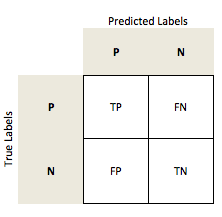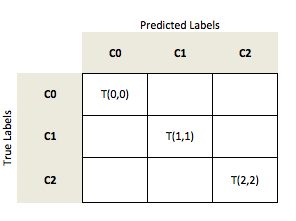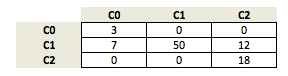# accuracy_score: Computing standard, balanced, and per-class accuracy

A function for computing for computing basic classifcation accuracy, per-class accuracy, and average per-class accuracy.

from mlxtend.evaluate import accuracy_score

## Example 1 -- Standard Accuracy

The "overall" accuracy is defined as the number of correct predictions (true positives TP and true negatives TN) over all samples n:

import numpy as np
from mlxtend.evaluate import accuracy_score

y_targ = [0, 0, 0, 1, 1, 1, 2, 2, 2]
y_pred = [1, 0, 0, 0, 1, 2, 0, 2, 2]

accuracy_score(y_targ, y_pred)

0.5555555555555556


## Example 2 -- Per-Class Accuracy

The per-class accuracy is the accuracy of one class (defined as the pos_label) versus all remaining datapoints in the dataset.

import numpy as np
from mlxtend.evaluate import accuracy_score

y_targ = [0, 0, 0, 1, 1, 1, 2, 2, 2]
y_pred = [1, 0, 0, 0, 1, 2, 0, 2, 2]

std_acc = accuracy_score(y_targ, y_pred)
bin_acc = accuracy_score(y_targ, y_pred, method='binary', pos_label=1)

print(f'Standard accuracy: {std_acc*100:.2f}%')
print(f'Class 1 accuracy: {bin_acc*100:.2f}%')

Standard accuracy: 55.56%
Class 1 accuracy: 66.67%


## Example 3 -- Average Per-Class Accuracy

#### Overview

The "overall" accuracy is defined as the number of correct predictions (true positives TP and true negatives TN) over all samples n:

in a binary class setting:In a multi-class setting, we can generalize the computation of the accuracy as the fraction of all true predictions (the diagonal) over all samples n.

Considering a multi-class problem with 3 classes (C0, C1, C2)let's assume our model made the following predictions:We compute the accuracy as:

Now, in order to compute the average per-class accuracy, we compute the binary accuracy for each class label separately; i.e., if class 1 is the positive class, class 0 and 2 are both considered the negative class.

import numpy as np
from mlxtend.evaluate import accuracy_score

y_targ = [0, 0, 0, 1, 1, 1, 2, 0, 0]
y_pred = [1, 0, 0, 0, 1, 2, 0, 2, 1]

std_acc = accuracy_score(y_targ, y_pred)
bin_acc = accuracy_score(y_targ, y_pred, method='binary', pos_label=1)
avg_acc = accuracy_score(y_targ, y_pred, method='average')

print(f'Standard accuracy: {std_acc*100:.2f}%')
print(f'Class 1 accuracy: {bin_acc*100:.2f}%')
print(f'Average per-class accuracy: {avg_acc*100:.2f}%')

Standard accuracy: 33.33%
Class 1 accuracy: 55.56%
Average per-class accuracy: 55.56%


## API

accuracy_score(y_target, y_predicted, method='standard', pos_label=1, normalize=True)

General accuracy function for supervised learning. Parameters

• y_target : array-like, shape=[n_values]

True class labels or target values.

• y_predicted : array-like, shape=[n_values]

Predicted class labels or target values.

• method : str, 'standard' by default.

The chosen method for accuracy computation. If set to 'standard', computes overall accuracy. If set to 'binary', computes accuracy for class pos_label. If set to 'average', computes average per-class (balanced) accuracy. If set to 'balanced', computes the scikit-learn-style balanced accuracy.

• pos_label : str or int, 1 by default.

The class whose accuracy score is to be reported. Used only when method is set to 'binary'

• normalize : bool, True by default.

If True, returns fraction of correctly classified samples. If False, returns number of correctly classified samples.

Returns

score: float

Examples

For usage examples, please see http://rasbt.github.io/mlxtend/user_guide/evaluate/accuracy_score/